# Chapter_12_Chemical_Kinetics.ppt

30 May 2023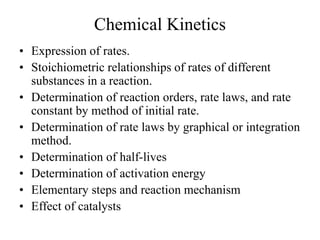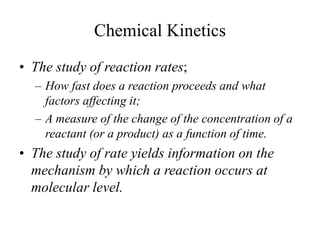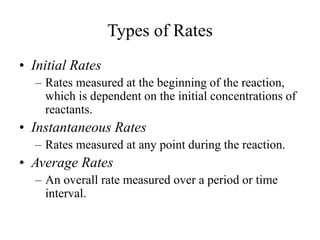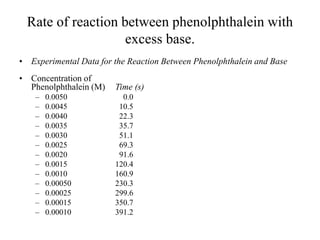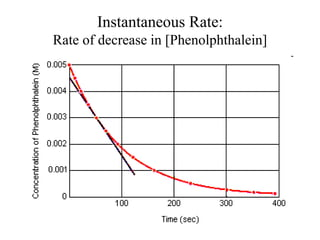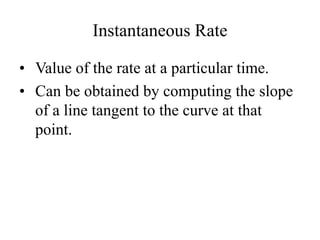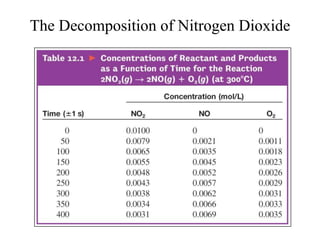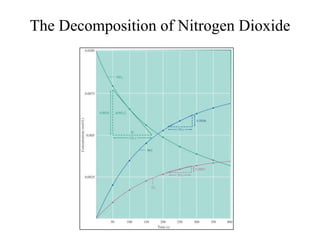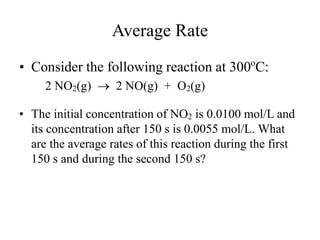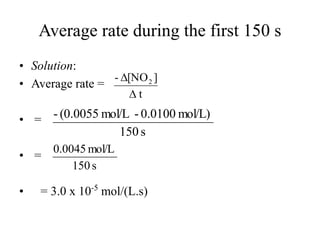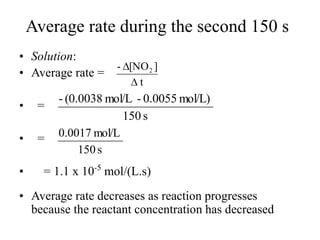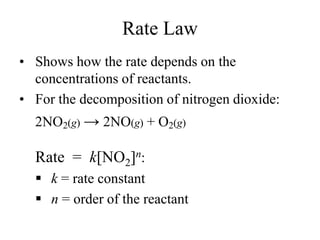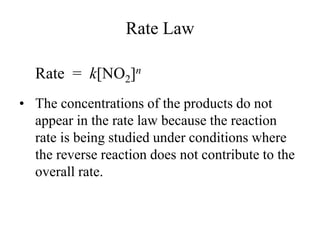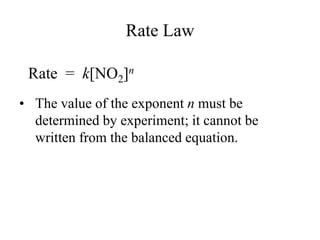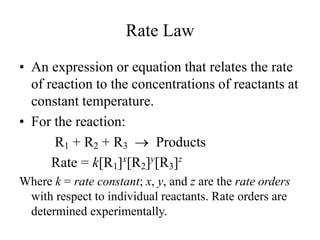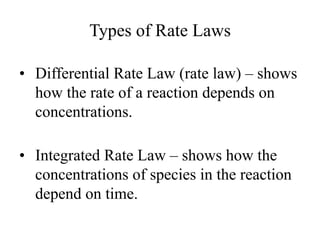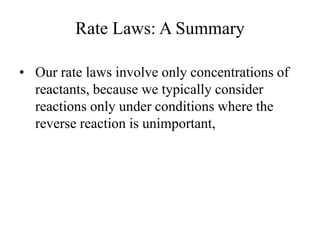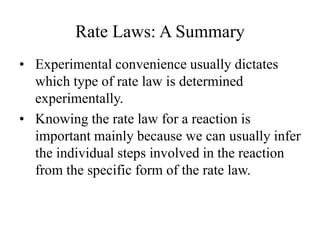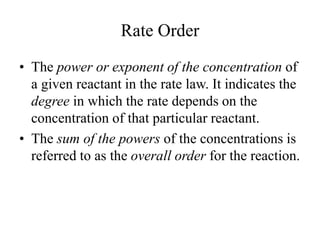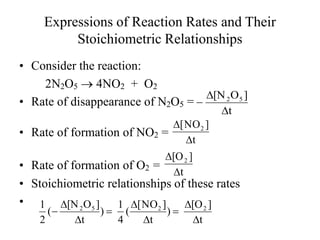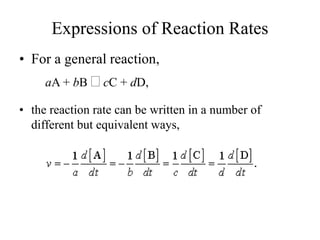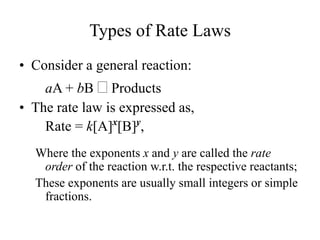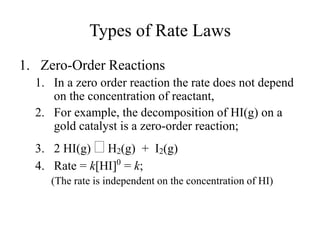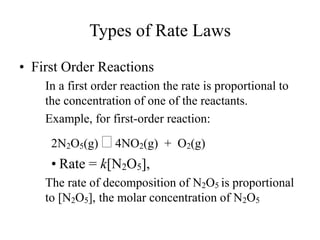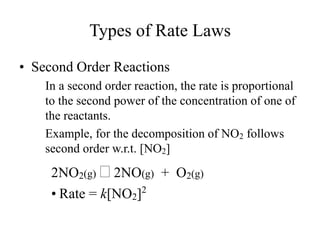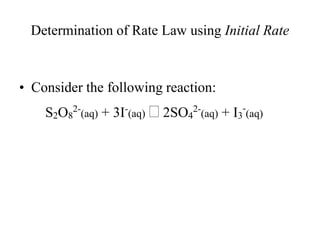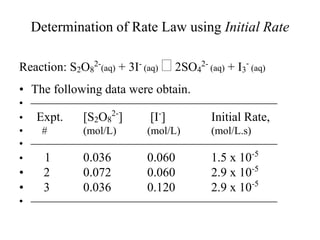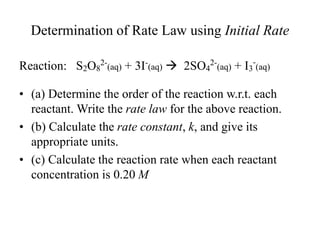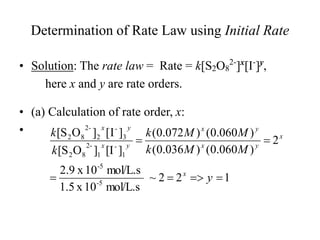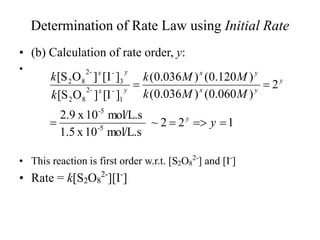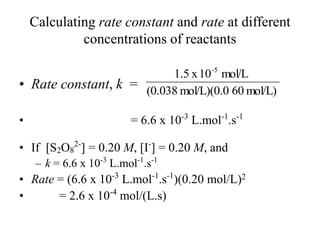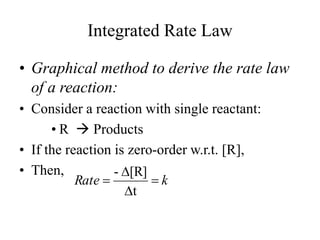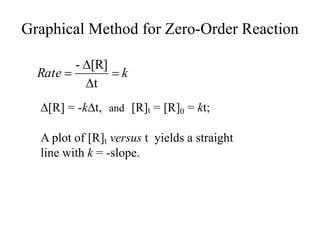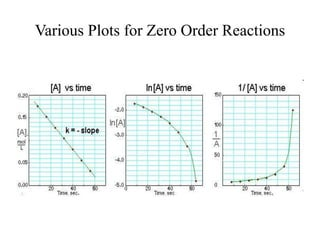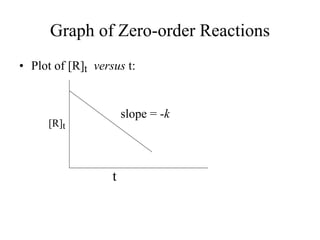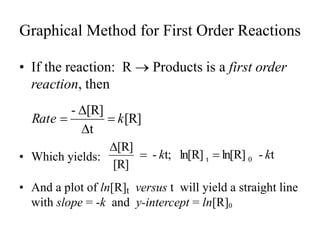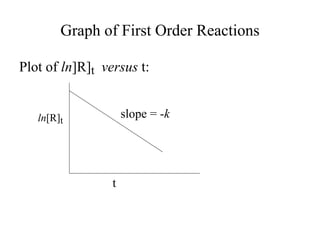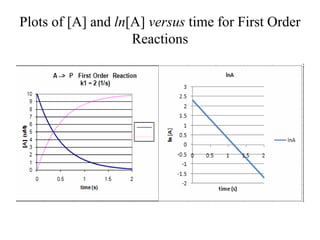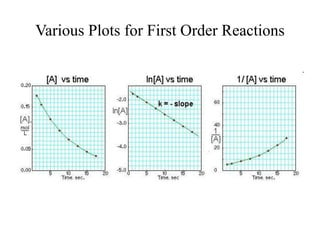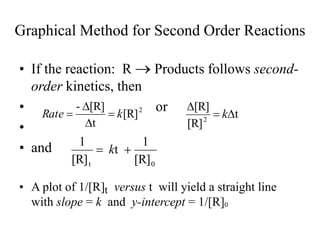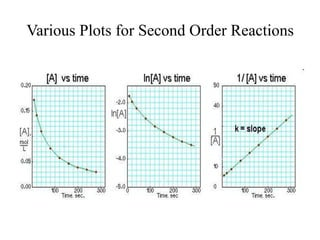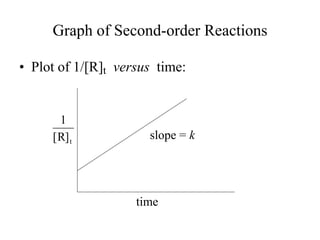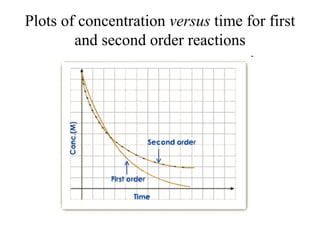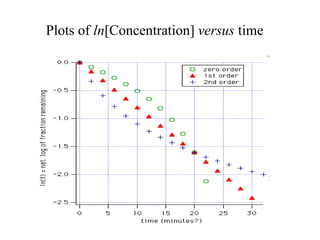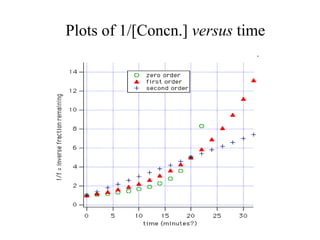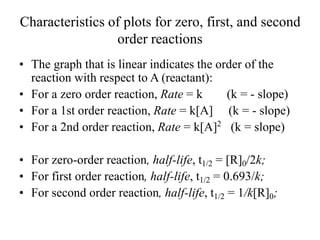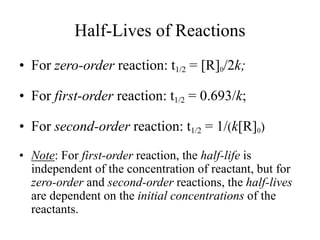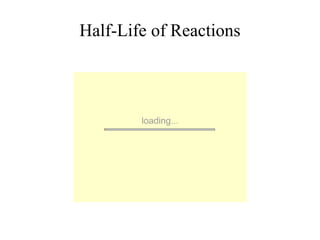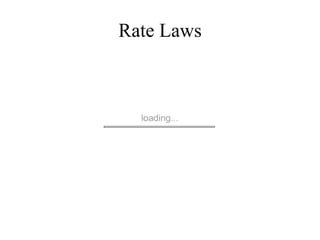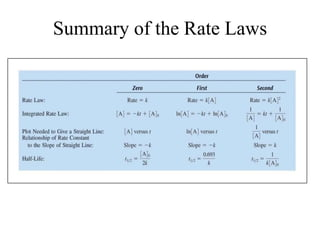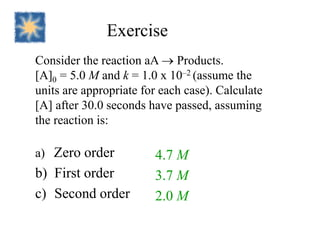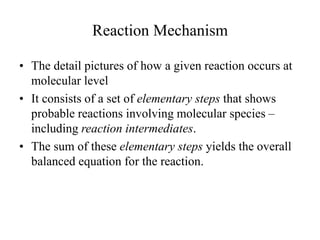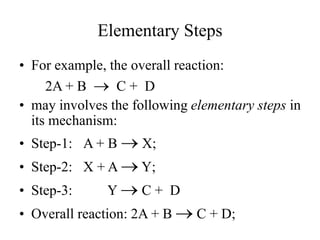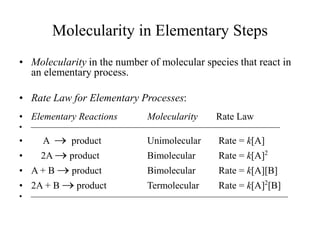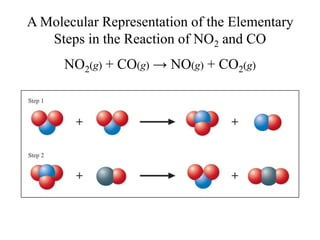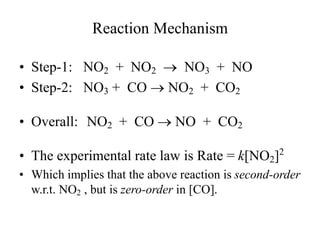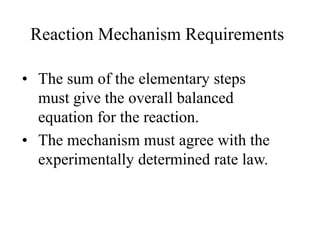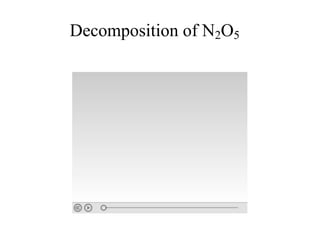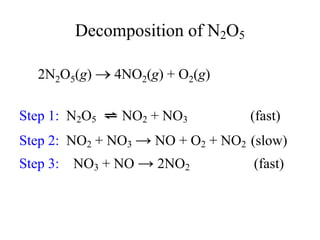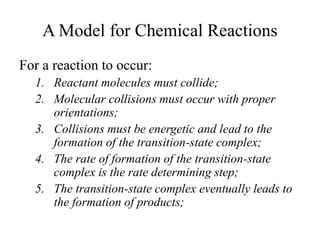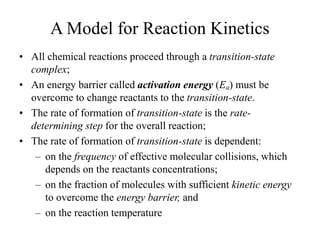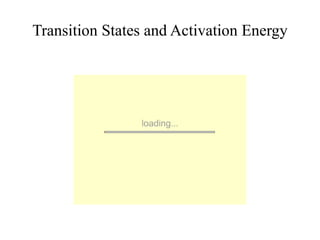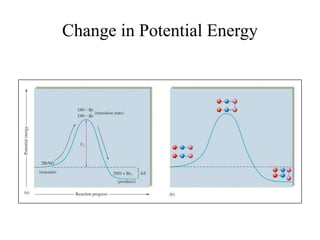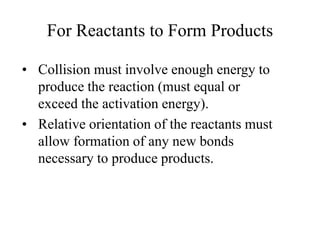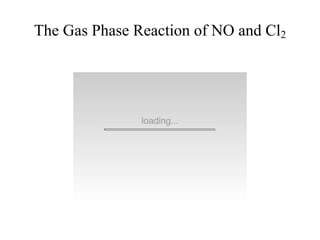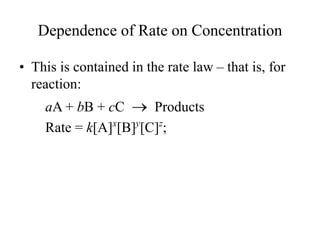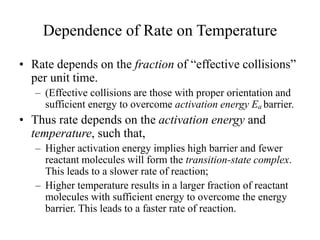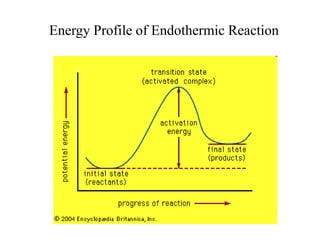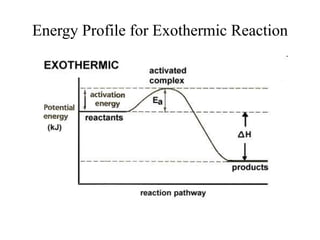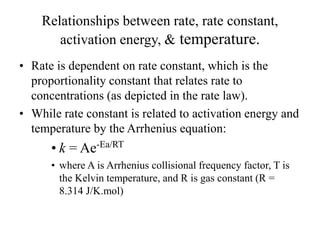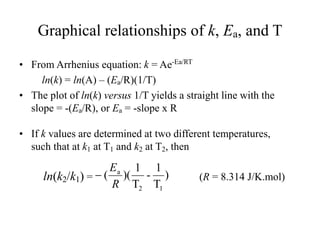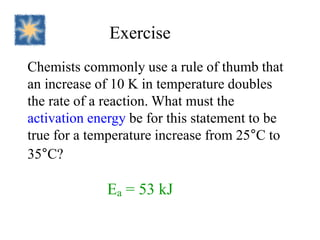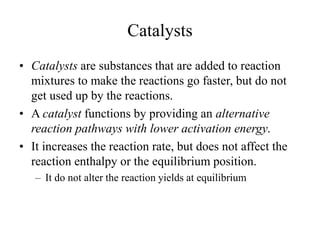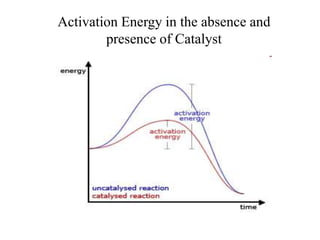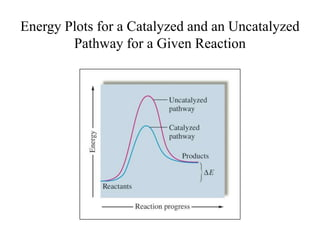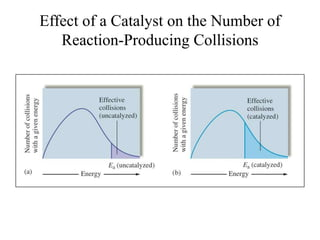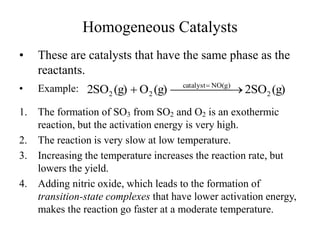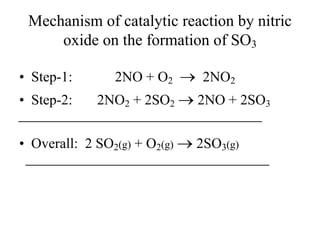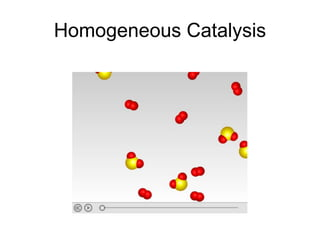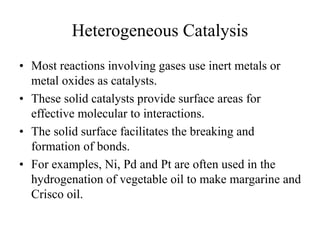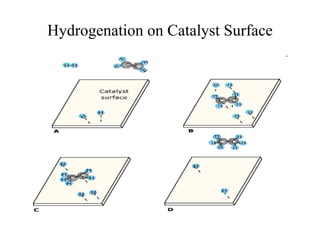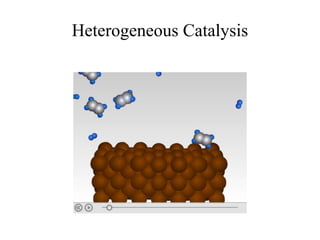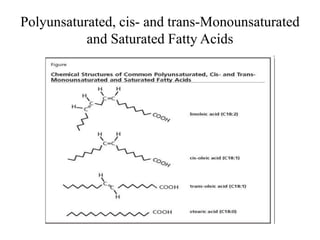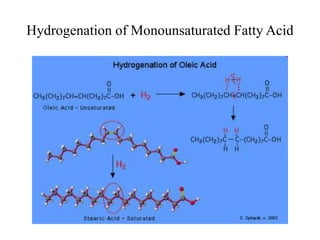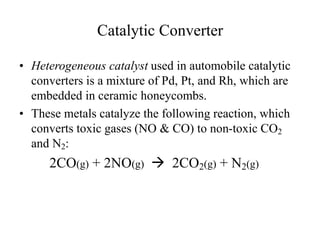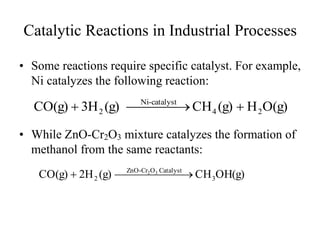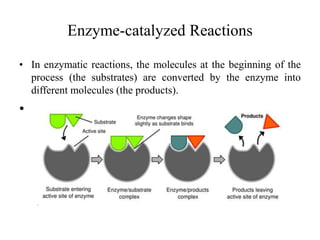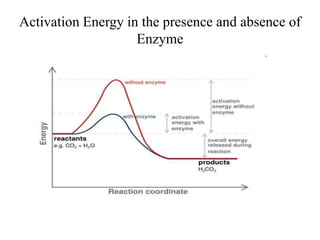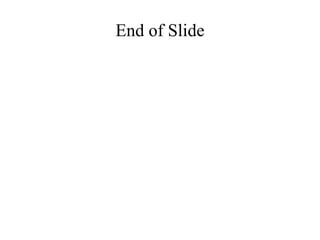1 sur 90

### Chapter_12_Chemical_Kinetics.ppt

• 1. Chemical Kinetics • Expression of rates. • Stoichiometric relationships of rates of different substances in a reaction. • Determination of reaction orders, rate laws, and rate constant by method of initial rate. • Determination of rate laws by graphical or integration method. • Determination of half-lives • Determination of activation energy • Elementary steps and reaction mechanism • Effect of catalysts
• 2. Chemical Kinetics • The study of reaction rates; – How fast does a reaction proceeds and what factors affecting it; – A measure of the change of the concentration of a reactant (or a product) as a function of time. • The study of rate yields information on the mechanism by which a reaction occurs at molecular level.
• 3. Types of Rates • Initial Rates – Rates measured at the beginning of the reaction, which is dependent on the initial concentrations of reactants. • Instantaneous Rates – Rates measured at any point during the reaction. • Average Rates – An overall rate measured over a period or time interval.
• 4. Rate of reaction between phenolphthalein with excess base. • Experimental Data for the Reaction Between Phenolphthalein and Base • Concentration of Phenolphthalein (M) Time (s) – 0.0050 0.0 – 0.0045 10.5 – 0.0040 22.3 – 0.0035 35.7 – 0.0030 51.1 – 0.0025 69.3 – 0.0020 91.6 – 0.0015 120.4 – 0.0010 160.9 – 0.00050 230.3 – 0.00025 299.6 – 0.00015 350.7 – 0.00010 391.2
• 5. Instantaneous Rate: Rate of decrease in [Phenolphthalein]
• 6. Instantaneous Rate • Value of the rate at a particular time. • Can be obtained by computing the slope of a line tangent to the curve at that point.
• 7. The Decomposition of Nitrogen Dioxide
• 8. The Decomposition of Nitrogen Dioxide
• 9. Average Rate • Consider the following reaction at 300o C: 2 NO2(g)  2 NO(g) + O2(g) • The initial concentration of NO2 is 0.0100 mol/L and its concentration after 150 s is 0.0055 mol/L. What are the average rates of this reaction during the first 150 s and during the second 150 s?
• 10. Average rate during the first 150 s • Solution: • Average rate = • = • = • = 3.0 x 10-5 mol/(L.s) t ] [NO - 2   s 150 mol/L) 0.0100 - mol/L (0.0055 - s 150 mol/L 0.0045
• 11. Average rate during the second 150 s • Solution: • Average rate = • = • = • = 1.1 x 10-5 mol/(L.s) • Average rate decreases as reaction progresses because the reactant concentration has decreased t ] [NO - 2   s 150 mol/L) 0.0055 - mol/L (0.0038 - s 150 mol/L 0.0017
• 12. Rate Law • Shows how the rate depends on the concentrations of reactants. • For the decomposition of nitrogen dioxide: 2NO2(g) → 2NO(g) + O2(g) Rate = k[NO2]n:  k = rate constant  n = order of the reactant
• 13. Rate Law Rate = k[NO2]n • The concentrations of the products do not appear in the rate law because the reaction rate is being studied under conditions where the reverse reaction does not contribute to the overall rate.
• 14. Rate Law Rate = k[NO2]n • The value of the exponent n must be determined by experiment; it cannot be written from the balanced equation.
• 15. Rate Law • An expression or equation that relates the rate of reaction to the concentrations of reactants at constant temperature. • For the reaction: R1 + R2 + R3  Products Rate = k[R1]x [R2]y [R3]z Where k = rate constant; x, y, and z are the rate orders with respect to individual reactants. Rate orders are determined experimentally.
• 16. Types of Rate Laws • Differential Rate Law (rate law) – shows how the rate of a reaction depends on concentrations. • Integrated Rate Law – shows how the concentrations of species in the reaction depend on time.
• 17. Rate Laws: A Summary • Our rate laws involve only concentrations of reactants, because we typically consider reactions only under conditions where the reverse reaction is unimportant,
• 18. Rate Laws: A Summary • Experimental convenience usually dictates which type of rate law is determined experimentally. • Knowing the rate law for a reaction is important mainly because we can usually infer the individual steps involved in the reaction from the specific form of the rate law.
• 19. Rate Order • The power or exponent of the concentration of a given reactant in the rate law. It indicates the degree in which the rate depends on the concentration of that particular reactant. • The sum of the powers of the concentrations is referred to as the overall order for the reaction.
• 20. Expressions of Reaction Rates and Their Stoichiometric Relationships • Consider the reaction: 2N2O5  4NO2 + O2 • Rate of disappearance of N2O5 = • Rate of formation of NO2 = • Rate of formation of O2 = • Stoichiometric relationships of these rates • t ] NO [ 2   t ] [O2   t ] O [N 5 2    t ] [O ) t ] NO [ ( 4 1 ) t ] O [N ( 2 1 2 2 5 2         
• 21. Expressions of Reaction Rates • For a general reaction, aA + bB  cC + dD, • the reaction rate can be written in a number of different but equivalent ways,
• 22. Rate Laws • For a general reaction, aA + bB + eE  Products • The rate law for this reaction takes the form: • where k is called the "rate constant." • x, y, and z, are small whole numbers or simple fractions and they are the rate order with respect to [A], [B], and [E]. The sum of x + y + z + . . . is called the “overall order" of the reaction.
• 23. Types of Rate Laws • Consider a general reaction: aA + bB  Products • The rate law is expressed as, Rate = k[A]x [B]y , Where the exponents x and y are called the rate order of the reaction w.r.t. the respective reactants; These exponents are usually small integers or simple fractions.
• 24. Types of Rate Laws 1. Zero-Order Reactions 1. In a zero order reaction the rate does not depend on the concentration of reactant, 2. For example, the decomposition of HI(g) on a gold catalyst is a zero-order reaction; 3. 2 HI(g)  H2(g) + I2(g) 4. Rate = k[HI]0 = k; (The rate is independent on the concentration of HI)
• 25. Types of Rate Laws • First Order Reactions In a first order reaction the rate is proportional to the concentration of one of the reactants. Example, for first-order reaction: 2N2O5(g)  4NO2(g) + O2(g) • Rate = k[N2O5], The rate of decomposition of N2O5 is proportional to [N2O5], the molar concentration of N2O5
• 26. Types of Rate Laws • Second Order Reactions In a second order reaction, the rate is proportional to the second power of the concentration of one of the reactants. Example, for the decomposition of NO2 follows second order w.r.t. [NO2] 2NO2(g)  2NO(g) + O2(g) • Rate = k[NO2]2
• 27. Determination of Rate Law using Initial Rate • Consider the following reaction: S2O8 2- (aq) + 3I- (aq)  2SO4 2- (aq) + I3 - (aq)
• 28. Determination of Rate Law using Initial Rate Reaction: S2O8 2- (aq) + 3I- (aq)  2SO4 2- (aq) + I3 - (aq) • The following data were obtain. •  • Expt. [S2O8 2- ] [I- ] Initial Rate, • # (mol/L) (mol/L) (mol/L.s) •  • 1 0.036 0.060 1.5 x 10-5 • 2 0.072 0.060 2.9 x 10-5 • 3 0.036 0.120 2.9 x 10-5 • 
• 29. Determination of Rate Law using Initial Rate Reaction: S2O8 2- (aq) + 3I- (aq)  2SO4 2- (aq) + I3 - (aq) • (a) Determine the order of the reaction w.r.t. each reactant. Write the rate law for the above reaction. • (b) Calculate the rate constant, k, and give its appropriate units. • (c) Calculate the reaction rate when each reactant concentration is 0.20 M
• 30. Determination of Rate Law using Initial Rate • Solution: The rate law = Rate = k[S2O8 2- ]x [I- ]y , here x and y are rate orders. • (a) Calculation of rate order, x: • 1 2 2 ~ mol/L.s 10 x 1.5 mol/L.s 10 x 2.9 2 ) 060 . 0 ( ) 036 . 0 ( ) 060 . 0 ( ) 072 . 0 ( ] [I ] O [S ] I [ ] O [S 5 - 5 - 1 - 1 - 2 8 2 3 - 2 - 2 8 2       y M M k M M k k k x x y x y x y x y x
• 31. Determination of Rate Law using Initial Rate • (b) Calculation of rate order, y: • • This reaction is first order w.r.t. [S2O8 2- ] and [I- ] • Rate = k[S2O8 2- ][I- ] 1 2 2 ~ mol/L.s 10 x 1.5 mol/L.s 10 x 2.9 2 ) 060 . 0 ( ) 036 . 0 ( ) 120 . 0 ( ) 036 . 0 ( ] [I ] O [S ] I [ ] O [S 5 - 5 - 1 - - 2 8 2 3 - - 2 8 2       y M M k M M k k k y y y x y x y x y x
• 32. Calculating rate constant and rate at different concentrations of reactants • Rate constant, k = • = 6.6 x 10-3 L.mol-1 .s-1 • If [S2O8 2- ] = 0.20 M, [I- ] = 0.20 M, and – k = 6.6 x 10-3 L.mol-1 .s-1 • Rate = (6.6 x 10-3 L.mol-1 .s-1 )(0.20 mol/L)2 • = 2.6 x 10-4 mol/(L.s) mol/L) 60 mol/L)(0.0 (0.038 mol/L 10 x 1.5 -5
• 33. Integrated Rate Law • Graphical method to derive the rate law of a reaction: • Consider a reaction with single reactant: • R  Products • If the reaction is zero-order w.r.t. [R], • Then, k Rate t [R] -    
• 34. Graphical Method for Zero-Order Reaction k Rate t [R] -     [R] = -kt, and [R]t = [R]0 = kt; A plot of [R]t versus t yields a straight line with k = -slope.
• 35. Various Plots for Zero Order Reactions
• 36. Graph of Zero-order Reactions • Plot of [R]t versus t: [R]t t slope = -k
• 37. Graphical Method for First Order Reactions • If the reaction: R  Products is a first order reaction, then • Which yields: • And a plot of ln[R]t versus t will yield a straight line with slope = -k and y-intercept = ln[R]0 [R] t [R] - k Rate     t - ln[R] ln[R] t; - [R] [R] 0 t k k   
• 38. Graph of First Order Reactions Plot of ln]R]t versus t: ln[R]t t slope = -k
• 39. Plots of [A] and ln[A] versus time for First Order Reactions
• 40. Various Plots for First Order Reactions
• 41. Graphical Method for Second Order Reactions • If the reaction: R  Products follows second- order kinetics, then • or • • and • A plot of 1/[R]t versus t will yield a straight line with slope = k and y-intercept = 1/[R]0 2 [R] t [R] - k Rate     t [R] [R] 2    k [R] 1 t [R] 1 0 t   k
• 42. Various Plots for Second Order Reactions
• 43. Graph of Second-order Reactions • Plot of 1/[R]t versus time: t R] [ 1 time slope = k
• 44. Plots of concentration versus time for first and second order reactions
• 45. Plots of ln[Concentration] versus time
• 46. Plots of 1/[Concn.] versus time
• 47. Characteristics of plots for zero, first, and second order reactions • The graph that is linear indicates the order of the reaction with respect to A (reactant): • For a zero order reaction, Rate = k (k = - slope) • For a 1st order reaction, Rate = k[A] (k = - slope) • For a 2nd order reaction, Rate = k[A]2 (k = slope) • For zero-order reaction, half-life, t1/2 = [R]0/2k; • For first order reaction, half-life, t1/2 = 0.693/k; • For second order reaction, half-life, t1/2 = 1/k[R]0;
• 48. Half-Lives of Reactions • For zero-order reaction: t1/2 = [R]0/2k; • For first-order reaction: t1/2 = 0.693/k; • For second-order reaction: t1/2 = 1/(k[R]0) • Note: For first-order reaction, the half-life is independent of the concentration of reactant, but for zero-order and second-order reactions, the half-lives are dependent on the initial concentrations of the reactants.
• 49. Half-Life of Reactions
• 50. Rate Laws
• 51. Summary of the Rate Laws
• 52. Exercise Consider the reaction aA  Products. [A]0 = 5.0 M and k = 1.0 x 10–2 (assume the units are appropriate for each case). Calculate [A] after 30.0 seconds have passed, assuming the reaction is: a) Zero order b) First order c) Second order 4.7 M 3.7 M 2.0 M
• 53. Reaction Mechanism • The detail pictures of how a given reaction occurs at molecular level • It consists of a set of elementary steps that shows probable reactions involving molecular species – including reaction intermediates. • The sum of these elementary steps yields the overall balanced equation for the reaction.
• 54. Elementary Steps • For example, the overall reaction: 2A + B  C + D • may involves the following elementary steps in its mechanism: • Step-1: A + B  X; • Step-2: X + A  Y; • Step-3: Y  C + D • Overall reaction: 2A + B  C + D;
• 55. Molecularity in Elementary Steps • Molecularity in the number of molecular species that react in an elementary process. • Rate Law for Elementary Processes: • Elementary Reactions Molecularity Rate Law •  • A  product Unimolecular Rate = k[A] • 2A  product Bimolecular Rate = k[A]2 • A + B  product Bimolecular Rate = k[A][B] • 2A + B  product Termolecular Rate = k[A]2 [B] • 
• 56. A Molecular Representation of the Elementary Steps in the Reaction of NO2 and CO NO2(g) + CO(g) → NO(g) + CO2(g)
• 57. Reaction Mechanism • Step-1: NO2 + NO2  NO3 + NO • Step-2: NO3 + CO  NO2 + CO2 • Overall: NO2 + CO  NO + CO2 • The experimental rate law is Rate = k[NO2]2 • Which implies that the above reaction is second-order w.r.t. NO2 , but is zero-order in [CO].
• 58. • The sum of the elementary steps must give the overall balanced equation for the reaction. • The mechanism must agree with the experimentally determined rate law. Reaction Mechanism Requirements
• 59. Decomposition of N2O5
• 60. Decomposition of N2O5 2N2O5(g)  4NO2(g) + O2(g) Step 1: N2O5 ⇌ NO2 + NO3 (fast) Step 2: NO2 + NO3 → NO + O2 + NO2 (slow) Step 3: NO3 + NO → 2NO2 (fast)
• 61. A Model for Chemical Reactions For a reaction to occur: 1. Reactant molecules must collide; 2. Molecular collisions must occur with proper orientations; 3. Collisions must be energetic and lead to the formation of the transition-state complex; 4. The rate of formation of the transition-state complex is the rate determining step; 5. The transition-state complex eventually leads to the formation of products;
• 62. A Model for Reaction Kinetics • All chemical reactions proceed through a transition-state complex; • An energy barrier called activation energy (Ea) must be overcome to change reactants to the transition-state. • The rate of formation of transition-state is the rate- determining step for the overall reaction; • The rate of formation of transition-state is dependent: – on the frequency of effective molecular collisions, which depends on the reactants concentrations; – on the fraction of molecules with sufficient kinetic energy to overcome the energy barrier, and – on the reaction temperature
• 63. Transition States and Activation Energy
• 64. Change in Potential Energy
• 65. • Collision must involve enough energy to produce the reaction (must equal or exceed the activation energy). • Relative orientation of the reactants must allow formation of any new bonds necessary to produce products. For Reactants to Form Products
• 66. The Gas Phase Reaction of NO and Cl2
• 67. Dependence of Rate on Concentration • This is contained in the rate law – that is, for reaction: aA + bB + cC  Products Rate = k[A]x [B]y [C]z ;
• 68. Dependence of Rate on Temperature • Rate depends on the fraction of “effective collisions” per unit time. – (Effective collisions are those with proper orientation and sufficient energy to overcome activation energy Ea barrier. • Thus rate depends on the activation energy and temperature, such that, – Higher activation energy implies high barrier and fewer reactant molecules will form the transition-state complex. This leads to a slower rate of reaction; – Higher temperature results in a larger fraction of reactant molecules with sufficient energy to overcome the energy barrier. This leads to a faster rate of reaction.
• 69. Energy Profile of Endothermic Reaction
• 70. Energy Profile for Exothermic Reaction
• 71. Relationships between rate, rate constant, activation energy, & temperature. • Rate is dependent on rate constant, which is the proportionality constant that relates rate to concentrations (as depicted in the rate law). • While rate constant is related to activation energy and temperature by the Arrhenius equation: • k = Ae-Ea/RT • where A is Arrhenius collisional frequency factor, T is the Kelvin temperature, and R is gas constant (R = 8.314 J/K.mol)
• 72. Graphical relationships of k, Ea, and T • From Arrhenius equation: k = Ae-Ea/RT ln(k) = ln(A) – (Ea/R)(1/T) • The plot of ln(k) versus 1/T yields a straight line with the slope = -(Ea/R), or Ea = -slope x R • If k values are determined at two different temperatures, such that at k1 at T1 and k2 at T2, then ln(k2/k1) = (R = 8.314 J/K.mol) ) T 1 - T 1 )( ( 1 2 a R E 
• 73. Exercise Chemists commonly use a rule of thumb that an increase of 10 K in temperature doubles the rate of a reaction. What must the activation energy be for this statement to be true for a temperature increase from 25°C to 35°C? Ea = 53 kJ
• 74. Catalysts • Catalysts are substances that are added to reaction mixtures to make the reactions go faster, but do not get used up by the reactions. • A catalyst functions by providing an alternative reaction pathways with lower activation energy. • It increases the reaction rate, but does not affect the reaction enthalpy or the equilibrium position. – It do not alter the reaction yields at equilibrium
• 75. Activation Energy in the absence and presence of Catalyst
• 76. Energy Plots for a Catalyzed and an Uncatalyzed Pathway for a Given Reaction
• 77. Effect of a Catalyst on the Number of Reaction-Producing Collisions
• 78. Homogeneous Catalysts • These are catalysts that have the same phase as the reactants. • Example: 1. The formation of SO3 from SO2 and O2 is an exothermic reaction, but the activation energy is very high. 2. The reaction is very slow at low temperature. 3. Increasing the temperature increases the reaction rate, but lowers the yield. 4. Adding nitric oxide, which leads to the formation of transition-state complexes that have lower activation energy, makes the reaction go faster at a moderate temperature. (g) 2SO (g) O (g) SO 2 2 NO(g) catalyst 2 2        
• 79. Mechanism of catalytic reaction by nitric oxide on the formation of SO3 • Step-1: 2NO + O2  2NO2 • Step-2: 2NO2 + 2SO2  2NO + 2SO3  • Overall: 2 SO2(g) + O2(g)  2SO3(g) 
• 80. Homogeneous Catalysis
• 81. Heterogeneous Catalysis • Most reactions involving gases use inert metals or metal oxides as catalysts. • These solid catalysts provide surface areas for effective molecular to interactions. • The solid surface facilitates the breaking and formation of bonds. • For examples, Ni, Pd and Pt are often used in the hydrogenation of vegetable oil to make margarine and Crisco oil.
• 82. Hydrogenation on Catalyst Surface
• 83. Heterogeneous Catalysis
• 84. Polyunsaturated, cis- and trans-Monounsaturated and Saturated Fatty Acids
• 85. Hydrogenation of Monounsaturated Fatty Acid
• 86. Catalytic Converter • Heterogeneous catalyst used in automobile catalytic converters is a mixture of Pd, Pt, and Rh, which are embedded in ceramic honeycombs. • These metals catalyze the following reaction, which converts toxic gases (NO & CO) to non-toxic CO2 and N2: 2CO(g) + 2NO(g)  2CO2(g) + N2(g)
• 87. Catalytic Reactions in Industrial Processes • Some reactions require specific catalyst. For example, Ni catalyzes the following reaction: • While ZnO-Cr2O3 mixture catalyzes the formation of methanol from the same reactants: O(g) H (g) CH (g) 3H CO(g) 2 4 catalyst - Ni 2        OH(g) CH (g) 2H CO(g) 3 Catalyst O Cr - ZnO 2 3 2        
• 88. Enzyme-catalyzed Reactions • In enzymatic reactions, the molecules at the beginning of the process (the substrates) are converted by the enzyme into different molecules (the products). •
• 89. Activation Energy in the presence and absence of Enzyme
• 90. End of Slide